# Algebra Worksheets

#### What is Algebra and How Is It Useful?

Algebra is a form math that helps us find unknown values which we refer to as variables. To represent variables, we often us symbols in the form of letter, for which the letter 'x' is the most commonly used. Early on algebraic equations are formed by means of basic arithmetic, but we can also understand patterns through the use of inequalities. This helps use recognize patters such as trends of increasing or decreasing, and or repeating patterns. When we take all this into account, we can use this form of math to all types of phenomena which will better help us understand the world around us. Where you can capture the attention of people, as far as the importance of algebra, is how useful algebra is for predicting or calculating financial based problems. In fact, many careers that focus on determining large values of money are foundationally based on algebra. In the field of science, we often find that the formulas can be manipulated and understood through the eyes of algebra.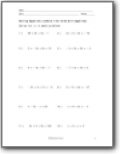###### Combine Like Terms

Solve for x using various methods.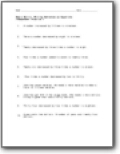###### Equations

Students will work with simple and complex equations that they will solve using several different techniques.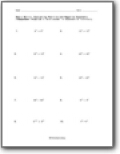###### Exponents

We work on a series of conversions between whole numbers and exponents.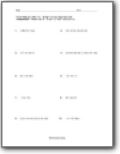###### Expressions

Explores working with and mastering expressions and manipulating them to serve you.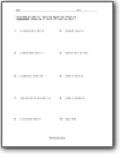###### Formulas

Students work on their evaluation skills to better understand formulas.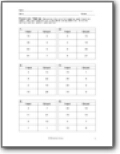###### Function Tables

A great introductory to algebra skill use.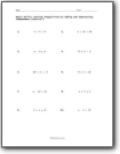###### Inequalities

Learn how to solve for unmet values and manipulate the use of inequalities.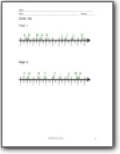###### Numbers Lines

Plotting numbers over various ranges.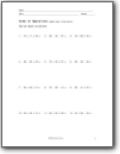###### Order of Operations

Students discover and practice PEMDAS operations. The process is unvailed.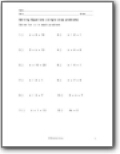###### Solve for x

Students jump through single, double, and triple step problems.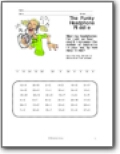###### Use of Symbols

Introduction to the use of numbers as symbols.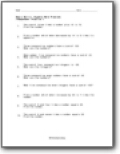###### Word Problems

These word problems are all based in unknowns and require your algebraic skills.